Tapping into mathematics

Start this free course now. Just create an account and sign in. Enrol and complete the course for a free statement of participation or digital badge if available.

Free course

# 4 Squares and other powers

Multiplying a number by itself is called squaring it and there is a key on scientific and graphics calculators which does this. On the TI-84 the key is marked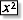.

## Exercise 4: Press some more

Try using the squaring key in the following key sequences. (If your calculator has an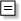key then use it instead of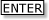).

• (a) 3• (b) 0.1• (c)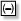4• (d)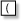4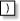• (e) 3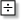2• (f)32Note any observations you have about the operation of the squaring key.

On the TI-84 you should get (a) 9, (b) .01, (c) 16, (d) 16, (e) .75, (f) 2.25

Unless there are brackets, thefunction operates only on the preceding number (without any sign) so in4the 4 is squared before theis applied. If you want to square 4, you need brackets.

Another way of saying ‘five squared’ is ‘five to the power 2’ and it is reasonable to extend this to other powers:

5 × 5 is written as 52 and is said ‘five to the power 2’

5 × 5 × 5 is written 53 and is said ‘five to the power 3’

5 × 5 × 5 × 5 is written 54 and is said ‘five to the power 4’ and so on.

There is a key on the calculator which can be used to carry out such calculations involving powers. It is usually marked as yx or ^. On the TI-84, it is the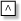key, and this key is on the right-hand side of the keyboard, above the operation keys.

To work out 2 × 2 × 2 × 2 × 2 or 25, for example, you press 25. Similarly 0.63 is the same as 0.6 × 0.6 × 0.6, which is 0.63 or 0.63. Try these now on your own calculator. Does it matter if you enter 0.6 or just .6?

You should get 25 is 32 and 0.63 is the same as .63 – they are both .216.

## Brain stretcher: Negative powers

What possible meaning could there be for negative powers, such as 7−3?

Try using the power key to work out the value of 2−1.

Does the answer look familiar? Try 2−2, 2−3, 2−4

Can you predict what 10−1, 10−2, 10−3 … might mean? Check your prediction on the calculator.

## Brain stretcher: Fractional powers

Press the following:

1 612What is going on here?

Press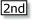[ENTRY] and change the 16 to other numbers such as 20, 25…

Once you know the effect of raising to the power, try other fractions such asand.

MU120_4MCAL